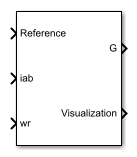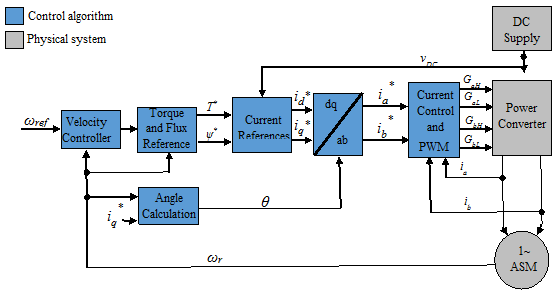# Induction Machine Field-Oriented Control (Single-Phase)

Per-unit discrete-time single-phase induction machine field-oriented control

Since R2018b

•Libraries:
Simscape / Electrical / Control / Induction Machine Control

## Description

The Induction Machine Field-Oriented Control (Single-Phase) block implements a single-phase induction machine field-oriented control structure.

### Equations

The field-oriented control architecture for a single-phase induction machine is:You can provide the torque reference as an input, or, in the case of speed control, generate the reference internally using a PI speed controller. The torque reference as derived from a PI speed controller is:

`$T*=\left({K}_{p_\omega }+{K}_{i_\omega }\frac{{T}_{s}z}{z-1}\right)\left({\omega }_{ref}-{\omega }_{r}\right)$`

where ωr is the rotor angular mechanical speed in rad/s.

The block generates the flux reference as

`$\psi *=\frac{2\pi {f}_{n}{\psi }_{n}}{p\mathrm{min}\left(|{\omega }_{r}|,\frac{2\pi {f}_{n}}{p}\right)}$`

where:

• p is the number of pole pairs.

• fn is the rated frequency.

• ψn is the rated flux.

Current references are obtained from machine parameters:

`${i}_{q}*=\frac{\left({L}_{ms}+{L}_{lar}\right)T*}{pa{L}_{ms}\psi *}$`

`${i}_{d}*=\frac{\psi *}{{a}^{2}{L}_{ms}}$`

where:

• Lms is the main winding mutual inductance.

• Llar is the main winding rotor leakage inductance.

• a is the auxiliary-to-main windings turn ratio.

The angle is calculated by solving:

`$\frac{d\theta }{dt}=p{\omega }_{r}+\frac{{a}^{3}{L}_{ms}{R}_{ar}}{{\psi }^{*}\left({L}_{ms}+{L}_{lar}\right)}$`

The transformation to the stationary reference frame is done by using:

`$\left(\begin{array}{c}{i}_{a}\\ {i}_{b}\end{array}\right)=\left(\begin{array}{cc}{a}^{2}\mathrm{sin}\left(\theta \right)& {a}^{2}\mathrm{cos}\left(\theta \right)\\ \mathrm{cos}\left(\theta \right)& -\mathrm{sin}\left(\theta \right)\end{array}\right)\left(\begin{array}{c}{i}_{d}\\ {i}_{q}\end{array}\right)$`

### Limitations

• The control structure is implemented in a single sample rate.

## Ports

### Input

expand all

Specify the angular velocity reference, in rad/s, or the torque reference, in Nm.

Data Types: `single` | `double`

Stator currents for main and auxiliary windings.

Data Types: `single` | `double`

Measured rotor angular speed, in rad/s.

Data Types: `single` | `double`

### Output

expand all

Inverter gate pulses, specified as a Boolean. The dead-time is not considered.

Data Types: `single` | `double`

Bus containing internal signals for visualization. The signals are:

• Reference torque or speed

• Stator currents (iab), in A

• Stator current reference (iabRef), in A

• Torque Reference (TqRef), in Nm

• Flux Reference (FluxRef), in Wb

Data Types: `single` | `double` | `bus`

## Parameters

expand all

### General

Specify the control mode. The reference input is taken as angular speed, in rad/s, for `Speed control` and torque, in Nm for `Torque control`.

Rated electrical frequency, in Hz.

Number of pole pairs.

Main winding rotor resistance, in Ohms.

Main winding rotor leakage inductance, in H.

Main winding mutual inductance, in H.

Auxiliary-to-main windings turns ratio.

Sample time for the block, in s.

Control sample time, in s.

### Outer Loop

This table shows how the visibility of some parameters in the Outer Loop tab depend on the option that you choose for the Control mode parameter in the General tab.

Control modeAvailable Parameters
Speed controlSpeed controller proportional gain
Speed controller integral gain
Speed controller integral anti-windup gain
Maximum torque (Nm)
Minimum torque (Nm)
Rated flux (Wb)
Torque controlRated flux (Wb)

Proportional gain for the PI speed controller.

Integral gain for the PI speed controller.

Integral anti-windup gain.

Maximum torque used in speed controller saturation, in Nm.

Minimum torque used in speed controller saturation, in Nm.

Rated flux, in Wb. The rated flux is used to compute the flux reference.

### Inner Loop

This table shows how the visibility of some parameters in the Inner Loop tab depend on the option that you choose for the Control type parameter.

Control typeAvailable Parameters
Hysteresis controlCurrent hysteresis band (A)
PI controlA-phase current proportional gain
A-phase current integral gain
A-phase current anti-windup gain
B-phase current proportional gain
B-phase current integral gain
B-phase current anti-windup gain

Control type for current control loop.

Current hysteresis band for the controller, in A.

Proportional gain for the A-phase (main winding) controller current.

Integral gain for the A-phase (main winding) controller current.

Anti-windup gain for the A-phase (main winding) controller current.

Proportional gain for the B-phase (auxiliary winding) controller current.

Integral gain for the B-phase (auxiliary winding) controller current.

Anti-windup gain for the B-phase (auxiliary winding) controller current.

 Correa, M. B. R., Jacobina, C. B., Lima, A. M. N., Da Silva, E. R. C. "Field Oriented Control of a Single-Phase Induction Motor Drive." PESC 98 Record. 29th Annual IEEE Power Electronics Specialists Conference. Vol. 2, 1998, pp. 990 - 996.